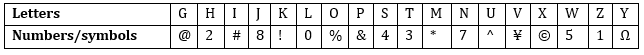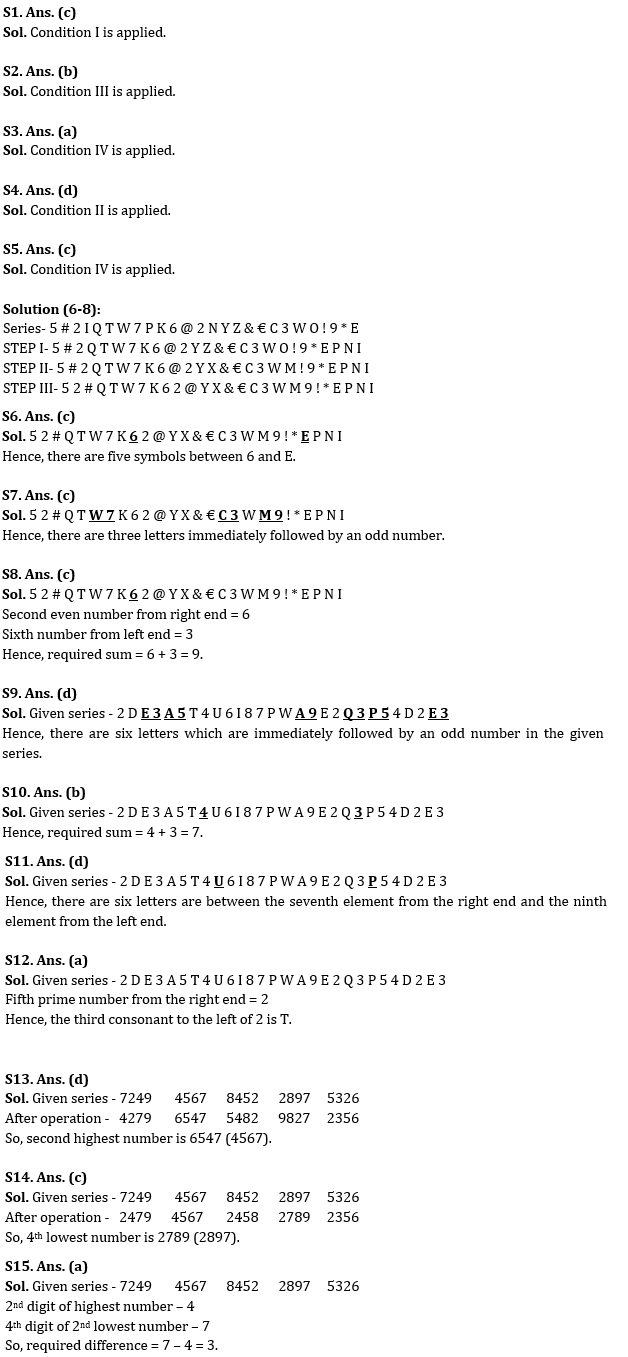Latest Banking jobs   »

# Reasoning Ability Quiz For IBPS RRB PO Clerk Mains 2022- 24th August

Directions (1-5): In each of the questions given below, a group of letters is given followed by some combinations of number/symbol. You have to find out which of given combination correctly represents the group of letters based on the numbers/symbols codes and the conditions given below. If none of the given combinations represents the group of letters correctly, give (e) i.e. ‘None of these’ as the answer.Condition for coding the group letters:
(i) If the first letter is vowel and last letter is consonant, the codes for the first and the last letter are to be interchanged.
(ii) If the first as well as the last letter is vowel, then both are to be coded by the code for the first letter.
(iii) If the first as well as the last letter is consonant, then both are to be coded by the code for the last letter.
(iv) If the first letter is consonant and last letter is vowel, the codes for the first and the last letter are to be coded as ®.

Q1. Find the code for ‘ILONXT’.
(e) None of these

Q2. Find the code for ‘SZGTUW’.
(a) 41@3^4
(b) 51@3^5
(c) 4@13^5
(d) 41@3^5
(e) None of these

Q3. Find the code for ‘JPWHNO’.
(a) ®&527®
(b) 8&527%
(c) 85&27®
(d) ®5&27®
(e) None of these

Q4. Find the code for ‘IMHYO’.
(a) #2* Ω#
(b) %*2 Ω%
(c) #*2 Ω%
(d) #*2 Ω#
(e) None of these

Q5. Find the code for ‘MVJPZI’.
(a) *8¥&1#
(b) *¥8&1#
(c) ®¥8&1®
(d) ®8&¥1®
(e) None of these

Directions (6-8): Study the following alphanumeric symbol series carefully and answer the questions given below.

5 # 2 I Q T W 7 P K 6 @ 2 N Y Z & € C 3 W O ! 9 * E

STEP I – The letters which are immediately preceded by number and immediately followed by a consonant are arranged just after E in the reverse alphabetical order in the above series.
STEP II – The letters which are immediately followed by symbol are replaced with their second preceding letter according to English alphabetical series.
STEP III – The symbol which is immediately followed by a number is interchanged its position with that number.
(STEP II is applied after STEP I and STEP III is applied after STEP II)

Q6. How many symbols are between 9th element from the left end and 4th element from right end in the last step?
(a) Two
(b) Three
(c) Five
(d) Six
(e) None of these

Q7. How many letters are immediately followed by an odd number in the last step?
(a) Two
(b) Four
(c) Three
(d) More than four
(e) None of these

Q8. What is the sum of the second even number from the right end and the sixth number from the left end in the last step?
(a) 6
(b) 7
(c) 9
(d) 8
(e) None of these

Directions (9-12): Read the given series of numbers and letters carefully and answer the questions based in it.

2 D E 3 A 5 T 4 U 6 I 8 7 P W A 9 E 2 Q 3 P 5 4 D 2 E 3

Q9. How many letters are immediately followed by an odd number?
(a) Three
(b) Four
(c) Five
(d) Six
(e) None of these

Q10. What is the sum of the third odd number from the right end and the fourth number from the left end?
(a) 9
(b) 7
(c) 10
(d) 16
(e) None of these

Q11. How many letters are in between the seventh element from the right end and the ninth element from the left end?
(a) Three
(b) Four
(c) Two
(d) More than four
(e) None of these

Q12. Which of the following is third consonant to the left of fifth prime number from the right end?
(a) T
(b) D
(c) W
(d) P
(e) None of these

Directions (13-15): Read the following four-digit number arrangement carefully and answer the questions based on it.

7249 4567 8452 2897 5326

Q13. If we interchange 1st and 3rd digit of each number, then which number will be second highest number?
(a) 7249
(b) 8452
(c) 2897
(d) 4567
(e) None of these

Q14. If we arrange all the digits of the numbers in the ascending order within the number, then which number will be the 4th lowest number after the rearrangement?
(a) 7249
(b) 8452
(c) 2897
(d) 4567
(e) None of these

Q15. What is the absolute difference between the 2nd digit of highest number and the 4th digit of 2nd lowest number in the given arrangement?
(a) 3
(b) 5
(c) 2
(d) 4
(e) None of these

Solutions#### Congratulations!Download Hindu Review of October 2021: Free PDF